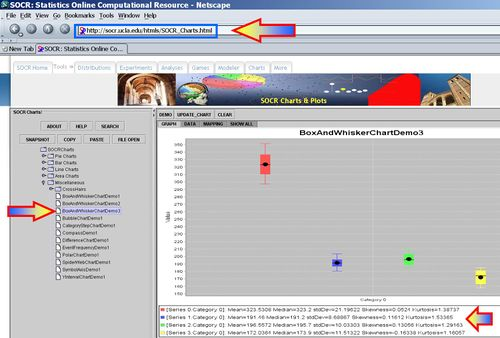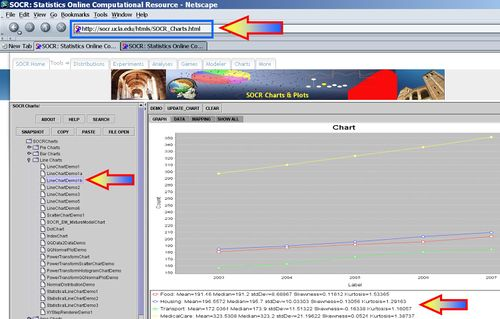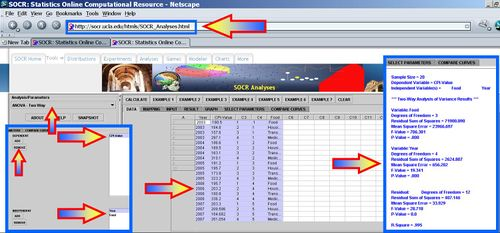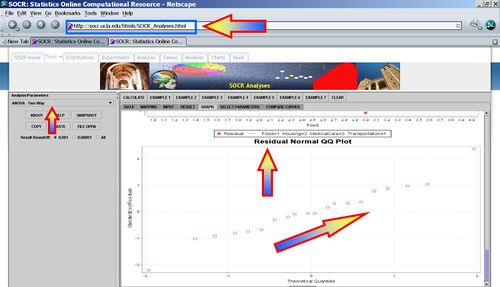# AP Statistics Curriculum 2007 ANOVA 2Way

## General Advance-Placement (AP) Statistics Curriculum - Two-Way Analysis of Variance (ANOVA)

In the previous section, we discussed statistical inference in comparing k independent samples separated by a single (grouping) factor. Now we will discuss variance decomposition of data into (independent/orthogonal) components when we have two (grouping) factors. Hence, this procedure called Two-Way Analysis of Variance.

### Motivational Example

Suppose we want to study the dynamics of the US Consumer Price Index (CPI), which is a major economic indicator that measures the average price changes of consumer goods and services.

To motivate 2-way ANOVA we can focus on a 5-year annual summary of the CPI for different types of consumer good (items). The complete dataset can be found here and a small 5-year excerpt is included below. Clearly, we have two-factors that may explain the dynamics of the CPI - year and item-type.

 Year CPI-Value Item-Code Year-Code Item 2003 180.5 1 1 Food 2003 184.8 2 1 Housing 2003 157.6 3 1 Transportation 2003 297.1 4 1 MedicalCare 2004 186.6 1 2 Food 2004 189.5 2 2 Housing 2004 163.1 3 2 Transportation 2004 310.1 4 2 MedicalCare 2005 191.2 1 3 Food 2005 195.7 2 3 Housing 2005 173.9 3 3 Transportation 2005 323.2 4 3 MedicalCare 2006 195.7 1 4 Food 2006 203.2 2 4 Housing 2006 180.9 3 4 Transportation 2006 336.2 4 4 MedicalCare 2007 203.3 1 5 Food 2007 209.586 2 5 Housing 2007 184.682 3 5 Transportation 2007 351.054 4 5 MedicalCare

 Year Food Housing Transportation MedicalCare 2003 180.5 184.8 157.6 297.1 2004 186.6 189.5 163.1 310.1 2005 191.2 195.7 173.9 323.2 2006 195.7 203.2 180.9 336.2 2007 203.3 209.586 184.682 351.054

Using the SOCR Charts (see SOCR Box-and-Whisker Plot Activity and Dot Plot Activity), we can generate plots that enable us to compare visually the 4 different CPI items (food, housing, transportation and medical-care) across the 5 years.We can also plot the time courses of these 4 CPI items (using the SOCR Line Chart.### Two-Way ANOVA Calculations

Let's make the following notation:

Two-way Model$y_{i,j,k} = \mu +\tau_i +\beta_j +\gamma_{i,j} + \epsilon_{i,j,k}$, for all $$1\leq i\leq a$$, $$1\leq j\leq b$$ and $$1\leq k\leq r$$. Here $$\mu$$ is the overall mean response, $$\tau_i$$ is the effect due to the $$i^{th}$$ level of factor A, $$\beta_j$$ is the effect due to the $$j^{th}$$ level of factor B and $$\gamma_{i,j}$$ is the effect due to any interaction between the $$i^{th}$$ level of factor A and the $$j^{th}$$ level of factor B.

$y_{i,j,k}$ = the A-factor level i and B-factor level j, observation-index k measurement.

k = number of replicates.

$a_i$ = number of A-factor observations at level i, $$a= a_1 + a_2 + \cdots + a_I$$. $b_j$ = number of B-factor observations at level j, $$b= b_1 + b_2 + \cdots + b_J$$.

N = total number of observations, $$N=a\times a \times b$$.
The mean of the A-factor group mean at level i and B-factor at level j is$\bar{y}_{i,j,.} = {\sum_{k=1}^{r}{y_{i,j,k}} \over r}$
The grand mean is$\bar{y}=\bar{y}_{.,.,.} = {\sum_{k=1}^r{\sum_{i=1}^a {\sum_{j=1}^{b}{y_{i,j,k}}}} \over N}$.

When an $$a \times b$$ factorial experiment is conducted with an equal number of observation per treatment combination, and where AB represents the interaction between A and B, the total (corrected) sum of squares is partitioned as:

$$SS(Total) = SS(A) + SS(B) + SS(AB) + SSE$$

### Hypotheses

There are three sets of hypotheses with the two-way ANOVA.

#### The null hypotheses for each of the sets

• The population means of the first factor are equal. This is like the one-way ANOVA for the row factor.
• The population means of the second factor are equal. This is like the one-way ANOVA for the column factor.
• There is no interaction between the two factors. This is similar to performing a test for independence with contingency tables.

#### Factors

The two independent variables in a two-way ANOVA are called factors (denoted by A and B). The idea is that there are two variables, factors, which affect the dependent variable (Y). Each factor will have two or more levels within it, and the degrees of freedom for each factor is one less than the number of levels.

#### Treatment Groups

Treatment Groups are formed by making all possible combinations of the two factors. For example, if the first factor has 5 levels and the second factor has 6 levels, then there will be $$5\times6=30$$ different treatment groups.

#### Main Effect

The main effect involves the independent variables one at a time. The interaction is ignored for this part. Just the rows or just the columns are used, not mixed. This is the part which is similar to the one-way analysis of variance. Each of the variances calculated to analyze the main effects is like the between variances

#### Interaction Effect

The interaction effect is the effect that one factor has on the other factor. The degree of freedom here is the product of the two degrees of freedom for each factor.

#### Within Variation

The Within variation is the sum of squares within each treatment group. You have one less than the sample size (remember all treatment groups must have the same sample size for a two-way ANOVA) for each treatment group. The total number of treatment groups is the product of the number of levels for each factor. The within variance is the within variation divided by its degrees of freedom. The within group is also called the error.

#### F-Tests

There is an F-test for each of the hypotheses, and the F-test is the mean square for each main effect and the interaction effect divided by the within variance. The numerator degrees of freedom come from each effect, and the denominator degrees of freedom is the degrees of freedom for the within variance in each case.

#### Two-Way ANOVA Table

It is assumed that main effect A has a levels (and df(A) = a-1), main effect B has b levels (and (df(B) = b-1), r is the sample size of each treatment, and $$N = a\times b\times n$$ is the total sample size. Notice the overall degree of freedom is once again one less than the total sample size.

 Variance Source Degrees of Freedom (df) Sum of Squares (SS) Mean Sum of Squares (MS) F-Statistics P-value Main Effect A df(A)=a-1 $$SS(A)=r\times b\times\sum_{i=1}^{a}{(\bar{y}_{i,.,.}-\bar{y})^2}$$ $${SS(A)\over df(A)}$$ $$F_o = {MS(A)\over MSE}$$ $$P(F_{(df(A), df(E))} > F_o)$$ Main Effect B df(B)=b-1 $$SS(B)=r\times a\times\sum_{j=1}^{b}{(\bar{y}_{., j,.}-\bar{y})^2}$$ $${SS(B)\over df(B)}$$ $$F_o = {MS(B)\over MSE}$$ $$P(F_{(df(B), df(E))} > F_o)$$ A vs.B Interaction df(AB)=(a-1)(b-1) $$SS(AB)=r\times \sum_{i=1}^{a}{\sum_{j=1}^{b}{((\bar{y}_{i, j,.}-\bar{y}_{i, .,.})+(\bar{y}_{., j,.}-\bar{y}))^2}}$$ $${SS(AB)\over df(AB)}$$ $$F_o = {MS(AB)\over MSE}$$ $$P(F_{(df(AB), df(E))} > F_o)$$ Error $$N-a\times b$$ $$SSE=\sum_{k=1}^r{\sum_{i=1}^{a}{\sum_{j=1}^{b}{(\bar{y}_{i, j,k}-\bar{y}_{i, j,.})^2}}}$$ $${SSE\over df(Error)}$$ Total N-1 $$SST=\sum_{k=1}^r{\sum_{i=1}^{a}{\sum_{j=1}^{b}{(\bar{y}_{i, j,k}-\bar{y}_{., .,.})^2}}}$$ ANOVA Activity

To compute the difference between the means, we will compare each group mean to the grand mean.

### SOCR ANOVA Calculations

SOCR Analyses provide the tools to compute the 2-way ANOVA. For example, the ANOVA for the Consumer Price Index data above may be easily computed - see the image below. Note that SOCR ANOVA requires the data to be entered in the column format of the first table in this section.Under the Graphs tab-pane, we can see a variety of plots demonstrating the ANOVA results.The ANOVA table for these data is reported under the Results tab-pane.

Sample Size = 20
Dependent Variable = CPI-Value
Independent Variable(s) = Year Item
--- Two-Way Analysis of Variance Results ---
Variable: Year
Degrees of Freedom = 4
Residual Sum of Squares = 2624.8073168000
Mean Square Error = 656.2018292000
F-Value = 19.3405175758
P-Value = .0000363212
Variable: Item
Degrees of Freedom = 3
Residual Sum of Squares = 71900.0898230001
Mean Square Error = 23966.6966076667
F-Value = 706.3807145121
P-Value = .0000000000
Residual: Degrees of Freedom = 12
Residual Sum of Squares = 407.1463920000
Mean Square Error = 33.9288660000
F-Value = 322.4822657900
P-Value = 0.0
R-Square = .9945664582

### Two-Way ANOVA Conditions

The Two-way ANOVA is valid if:

• The populations from which the samples were obtained must be normally or approximately normally distributed.
• The samples must be independent.
• The variances of the populations must be equal.
• The groups must have the same sample size.

### Clinical example: Knee Pain Study

The following data was collected in a large knee pain study (N=6,046) by TMT Medical and UCLA investigators using an online questionnaire. The goals of the study was to identify the relations between a large number of clinical markers, patient phenotypes and imaging data of knee pain. One specific set of research questions the investigators were interested in related to the effects of mild treatment by applying hot or cold patches to the affected knee areas. The 2 tables below present identical information, however, the second table may be used to paste in the data into the SOCR 2-way ANOVA Applet or any other SOCR analysis tool. Try to answer 2 types of questions:

• Are there significant effects of the type-of-diagnosis (DiagnosisType) and/or the type-of-treatment (TreatmentType(Cold=1_Hot=2)) on the observed frequencies of reported benefits?
• Are there interactions between the type-of-diagnosis and the type-of-treatment?
Diagnosis RespondersToColdTreatment RespondersToHotTreatment NoResponseToHotOrColdTreatment Total
A_Bursitis 18 3 32 53
I_Bursitis 14 1 13 28
P_Bursitis 33 17 78 128
LCL_Injury 10 6 39 55
MCL_Injury 56 22 95 173
PCL_Injury 6 4 17 27
Bakers_Cyst 28 15 91 134
Chondromalacia 290 90 478 858
Dislocation 37 8 102 147
IBS 173 29 262 464
Lateral_Meniscus 49 10 110 169
Medial_Meniscus 147 35 277 459
Osteoarthritis 442 290 1478 2210
Other 102 57 337 496
Plica_Syndrome 24 9 33 66
Rheumatoid_Arthritis 25 19 115 159
Tendon_Tear 25 7 61 93
ClinicalDiagnosis DiagnosisType TreatmentType(Cold=1_Hot=2) NumberOfRespondersToTreatment
ACL_Injury Acute_Injury 1 110
Dislocation Acute_Injury 1 37
Lateral_Meniscus Acute_Injury 1 49
LCL_Injury Acute_Injury 1 10
MCL_Injury Acute_Injury 1 56
Medial_Meniscus Acute_Injury 1 147
PCL_Injury Acute_Injury 1 6
Tendon_Tear Acute_Injury 1 25
Osteoarthritis Arthritis 1 442
Rheumatoid_Arthritis Arthritis 1 25
A_Bursitis Bursitis 1 18
I_Bursitis Bursitis 1 14
P_Bursitis Bursitis 1 33
Plica_Syndrome Other 1 24
Chondromalacia Overuse 1 290
IBS Overuse 1 173
Bakers_Cyst Other 1 28
Other Other 1 102
ACL_Injury Acute_Injury 2 18
Dislocation Acute_Injury 2 8
Lateral_Meniscus Acute_Injury 2 10
LCL_Injury Acute_Injury 2 6
MCL_Injury Acute_Injury 2 22
Medial_Meniscus Acute_Injury 2 35
PCL_Injury Acute_Injury 2 4
Tendon_Tear Acute_Injury 2 7
Osteoarthritis Arthritis 2 290
Rheumatoid_Arthritis Arthritis 2 19
A_Bursitis Bursitis 2 3
I_Bursitis Bursitis 2 1
P_Bursitis Bursitis 2 17
Plica_Syndrome Other 2 9
Chondromalacia Overuse 2 90
IBS Overuse 2 29
Bakers_Cyst Other 2 15
Other Other 2 57